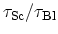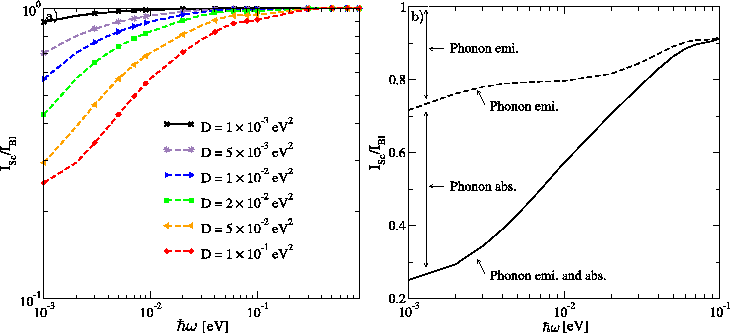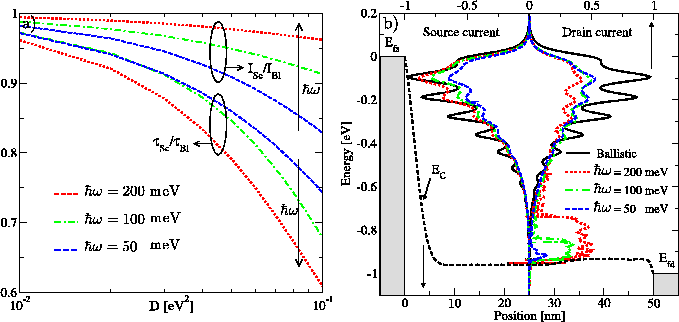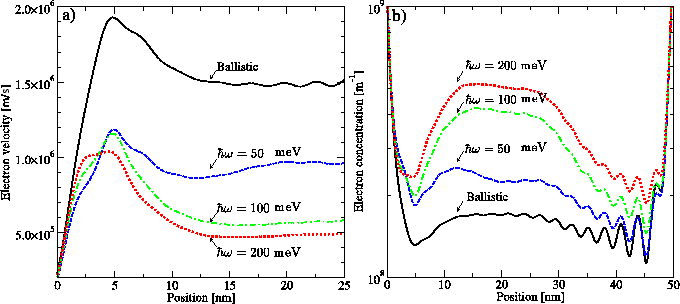## 5.5.2 Phonon Energy

Figure 5.22-a shows the dependence of the ballisticity with respect to the phonon energy. With increasing phonon energy the effect of phonon scattering on the current is reduced, because scattered electrons lose more kinetic energy and the probability for traveling back to the source contact decreases. The considerable decrease of ballisticity for low energy phonons is due to the phonon absorption process.

The right part of Fig. 5.20-b shows an electron absorbing energy from a phonon and scattering into a higher energy state. In this case, the probability for arriving at the source contact increases. This process can severely reduce the total current.

Fig. 5.22-b separately shows the effects of the phonon emission and absorption processes on the ballisticity. As the phonon energy decreases, the phonon occupation number (C.18) increases exponentially, and the self-energy contributions of these two components increase. However, due to the higher probability for back-scattering of electrons in the case of phonon absorption, this component reduces the total current more effectively than the phonon emission process does.

Fig. 5.23-a shows the ratio of the gate-delay time (Section 5.3.1) in the presence of electron-phonon interaction to that in the ballistic case,, as a function of the electron-phonon coupling strength. As the phonon energy increases the gate-delay time increases. This behavior can be attributed to the average electron velocity in the channel, which is high for ballistic electrons and low for electrons scattered to lower energy states.Fig. 5.23-b shows the spectra of the source and drain currents for different inelastic phonon energies. Electrons can emit a single phonon or a couple of phonons to reach lower energy states. The probability of multiple phonon emissions decreases as the number of interactions increases. Therefore, as the phonon energy increases, the occupation of electrons at lower energy states increases.

As shown in Fig. 5.23-b, the electron population close to the conduction band-edge considerably increases as the phonon energy increases. Therefore, as the phonon energy increases the mean velocity of electrons decreases and the carrier concentration in the channel increases (Fig. 5.24). The increased charge in the channel results in an increased gate-delay time.

M. Pourfath: Numerical Study of Quantum Transport in Carbon Nanotube-Based Transistors